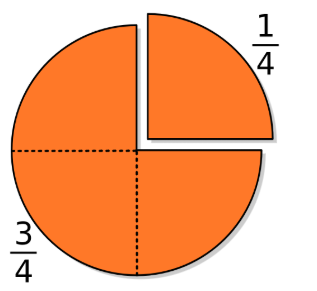# Equal Fractions

Computational Thinking### Overview​

The Fraction Equality Lab allows students to experiment with fractions by creating equivalent fractions. Different shapes are used as well as a number line. When the game is played students select a level and match equivalent fractions. The higher the level the more challenging it will be.

Students will:

•  Be able to understand fractions as equivalent, recognize and generate equivalent fractions, and express whole numbers as fractions as they use the Equal Fractions Lab and Game.

Vocabulary Words:

1. Fraction: A fraction is a part of a whole.
2. Numerator: A numerator is a number above the line of a fraction.
3. Denominator: A denominator is a number below the line of a fraction.
4. Equivalent Fraction: An equivalent fraction is different fractions that represent the same number.
5. Improper Fraction: An improper fraction is a fraction in which the numerator is greater than the denominator, such as 5/4.

To prepare for this lesson:

• The teacher should introduce the students to fractions. Prior knowledge is helpful.

• The teacher will preview the Equality Fraction lab

• The teacher should preview the levels to be sure of the appropriateness for the student.

• Check out the following videos:

See Accommodations Page and Charts on the 21things4students.net site in the Teacher Resources.

Directions for this activity:

1. Students go to Fractions: Equality.
2. Begin in the Equality Lab.
3. Select the desired shape on the top left toolbar.
4. Select if you want to use the same shape or a number line to compare the fractions.
5. Drag pieces from the bucket to the fraction board.
6. Compare the fractions.
7. To Play the game go back to the home page and click on Game.
8. Select the level to play. Recommend to begin at Level One.
9. Drag equivalent fractions to the scale.
10. Check.
11. Click OK and continue.

Note: Use any of the videos with the students before introducing the activity.

Different options for assessing the students:

• Observations
• Check for understanding
• Math Talks
• Number Talks
• Teacher Observations
• Exit Tickets
• Teacher assessments
• Equivalent Fractions Assessment

MITECS: Michigan adopted the "ISTE Standards for Students" called MITECS (Michigan Integrated Technology Competencies for Students) in 2018.

Computational Thinking
5a. Students formulate problem definitions suited for technology-assisted methods such as data analysis, abstract models and algorithmic thinking in exploring and finding solutions.
5b. Students collect data or identify relevant data sets, use digital tools to analyze them, and represent data in various ways to facilitate problem-solving and decision-making.
5c. Students break problems into component parts, extract key information, and develop descriptive models to understand complex systems or facilitate problem-solving.
5d. Students understand how automation works and use algorithmic thinking to develop a sequence of steps to create and test automated solutions.

Browser: Chrome, Safari, Firefox, Edge, ALL

Websites:
Fractions: Equality